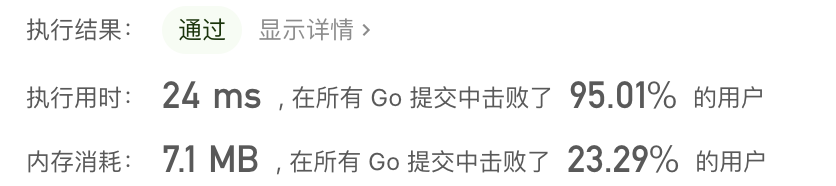|     |   0 浏览

## 题目描述

``````输入两棵二叉树A和B，判断B是不是A的子结构。(约定空树不是任意一个树的子结构)

B是A的子结构， 即 A中有出现和B相同的结构和节点值。

3
/ \
4   5
/ \
1   2

4
/
1

0 <= 节点个数 <= 10000
``````

## 解法

go代码如下：

``````func isSubStructure(A *TreeNode, B *TreeNode) bool {
if A == nil || B == nil {
return false
}

return dfs(A, B) || isSubStructure(A.Left, B) || isSubStructure(A.Right, B)
}

func dfs(A *TreeNode, B * TreeNode) bool {
if B == nil {
return true
}
if A == nil {
return false
}
return A.Val == B.Val && dfs(A.Left, B.Left) && dfs(A.Right, B.Right)
}
``````